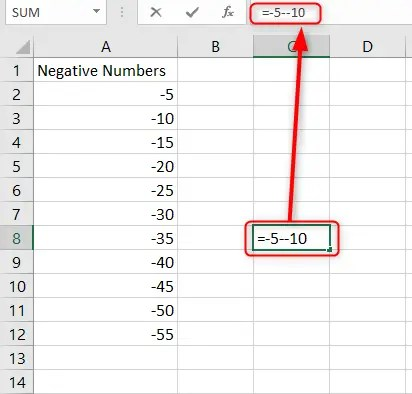# How to subtract negative numbers in Excel

Microsoft Excel is a powerful tool that allows businesses and organizations to calculate complex mathematical equations and create intricate formulas. Subtraction is among the basic arithmetic operations in Excel. For instance, you can easily calculate the difference between multiple negative numbers and positive numbers. You can subtract the manual way in Excel by using the SUM function.

In this article, we explain t how you can subtract negative numbers in Excel. Let’s get started.

## Normal subtraction in Excel

Computing the differences between negative numbers using normal subtraction is easy.

1. In an open Excel worksheet, click on a cell that is empty where you want your results displayed.

2. Type an = symbol followed by the numbers you wish to minus.

3. Enter any numbers to minus at the same time. (It doesn’t matter whether its positive or negative.)

4. Use the minus sign before your negative numbers and when subtracting.

5. Press Enter.Note:

This method can be time-consuming as you have to write a formula for every set of numbers you have individually.

## Subtracting using cell references in Excel

You can subtract your negative values in Excel while referencing the cells instead of in a single cell. It is a more effective and time-saving method as you can apply the same formula to multiple cells within your workbook.

1. In an open Excel workbook, click on an empty cell where you want your results displayed.

2. Press the equal sign first (=).

3. Click on the first cell.

4. On your keyboard hit the subtractions symbol (-) and then click the next cell to include it in your computation.5. Do this for all the cells and then press the Enter key.

## Subtracting a range with negative values using the ABS function

The ABS function enables you to get the absolute difference between two numbers in Excel workbooks. The ABS function will return an absolute value of a number which means a number without its sign. The formula for the ABS function is:

### ABS (number)

To use this formula, here is what you will do:

1. In an open Excel workbook, click on an empty cell where you wish to display your results.

2. In these cells, type the equal sign (=) followed by the ABS formula. In our case, it will be =ABS(

3. Click on your Minued cell (the first cell with the figure to subtract)

4. Next, put a minus sign followed by the subtrahend value.

5. Close the bracket on the formula and click the Enter key.6. Do this for all the cells. Use the AutoFill handle to apply your formula to the other cells.

### Subtracting a range of negative numbers

When you have several cells that you want to subtract, it can be tiresome to use the above two methods. You may need an easier process and method to subtract all the cells at a go and avoid the risk of mathematical errors. You can achieve this by using the SUM formula in Excel. The SUM function allows you to calculate a range of cells at a go.